# Replace values of a DataFrame with the value of another DataFrame in Pandas

In this article, we will learn how we can replace values of a DataFrame with the value of another DataFrame using pandas.

It can be done using the DataFrame.replace() method. It is used to replace a regex, string,  list, series, number, dictionary, etc. from a DataFrame, Values of the DataFrame method are get replaced with another value dynamically. This is a very rich function as it has many methods. This tutorial explains different examples of how to use these methods in practice.

Syntax: DataFrame.replace( old value, new value)

Parameters:

• old value: This is old substring to be replaced.
• new value: This is new substring, which would replace old substring.

### Examples

Here we will create some data that we will use in further examples.

## Python3

 `import` `pandas as pd`     `# initialise data of lists.` `colors ``=` `{``'first_set'``:  [``'99'``, ``'88'``, ``'77'``, ``'66'``,` `                         ``'55'``, ``'44'``, ``'33'``, ``'22'``],` `          ``'second_set'``: [``'1'``, ``'2'``, ``'3'``, ``'4'``, ``'5'``,` `                         ``'6'``, ``'7'``, ``'8'``]` `          ``}`   `color ``=` `{``'first_set'``:  [``'a'``, ``'b'``, ``'c'``, ``'d'``, ``'e'``,` `                        ``'f'``, ``'g'``, ``'h'``],` `         ``'second_set'``: [``'VI'``, ``'IN'``, ``'BL'``, ``'GR'``,` `                        ``'YE'``, ``'OR'``, ``'RE'``, ``'WI'``]` `         ``}` `# Calling DataFrame constructor on list` `df ``=` `pd.DataFrame(colors, columns``=``[``'first_set'``, ``'second_set'``])` `df1 ``=` `pd.DataFrame(color, columns``=``[``'first_set'``, ``'second_set'``])`   `# Display the Output` `display(df)` `display(df1)`

Output: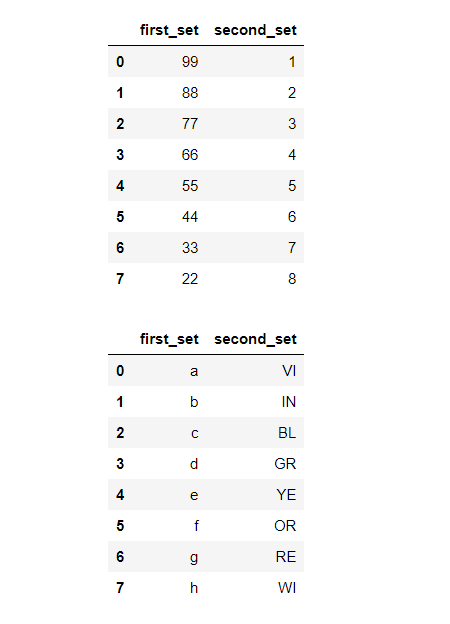Example 1: Replace the “e”  value with the “88” value of first_set of a DataFrame.

## Python3

 `# selecting old value` `a ``=` `df1[``'first_set'``][``4``] `   `# selecting new value` `b ``=` `df[``'first_set'``][``1``]`     `# replace values of one DataFrame ` `# with the value of another DataFrame` `df1 ``=` `df1.replace(a,b)`   `# Display the Output` `display(df1)`

Output: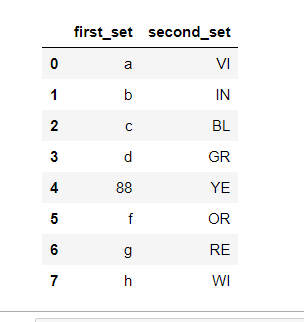Example 2: Replace the “55”  value with the “b” value of first_setof a DataFrame.

## Python3

 `# Display the Output` `display(df)` `display(df1)`   `# Selecting old value` `a ``=` `df[``'first_set'``][``4``] `   `# Selecting new value` `b ``=` `df1[``'first_set'``][``1``]`   `# replace values of one DataFrame with` `# the value of another DataFrame` `df ``=` `df.replace(a,b)`   `# Display the Output` `display(df)`

Output: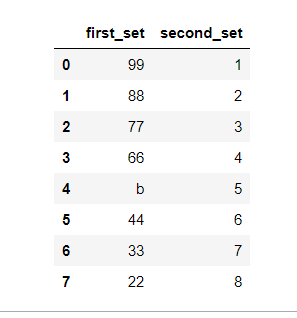Example 3: Now let’s replace the ’55’ values with ‘Hello’ values under the ‘first_set’ column and the ‘VI’ values with ‘Geeks’ values under the second_set’ column of a DataFrame.

## Python3

 `#selected value` `a ``=` `df[``'first_set'``][``4``] ` `b ``=` `df1[``'second_set'``][``1``]`   `# replace values of one DataFrame with ` `# the value of another DataFrame` `df ``=` `df.replace(a,``'Hello'``)`   `# replace values of one DataFrame with ` `# the value of another DataFrame` `df1 ``=` `df1.replace(b,``'Geeks'``)`     `display (df)` `display(df1)`

Output: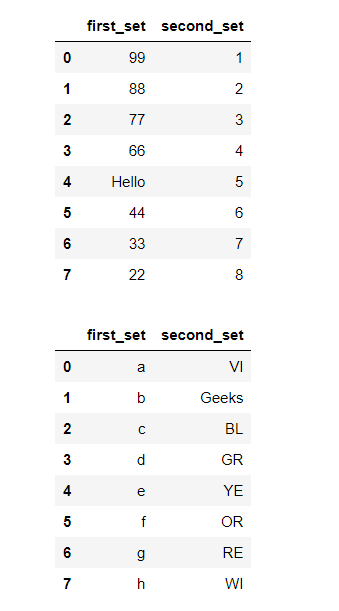Example 4: Let’s now replace the whole column of one DataFrame with the column of another DataFrame.

## Python3

 `# replace column of one DataFrame with ` `# the column of another DataFrame` `df[``'second_set'``] ``=` `df1.replace(df[``'first_set'``],df[``'second_set'``])`   `display(df)`

Output: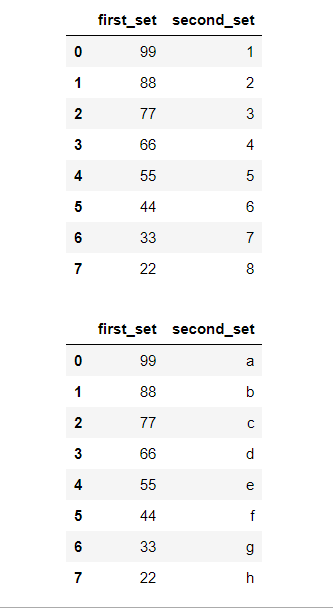Example 5: Here, df1.first_set[df1.first_set == ’66’] = ‘DF1’ means that firstly we are finding ’66’ in first_set of df1, after that we are replacing that value (’66’) with ‘DF1’ for the same position.

## Python3

 `# replacing value of DataFrame` `df1.first_set[df1.first_set ``=``=` `'66'``] ``=` `'DF1'` `df2.first_set[df2.first_set ``=``=` `'g'``] ``=` `'DF2'`   `# display updated table` `display(df1)` `display(df2)`

Output: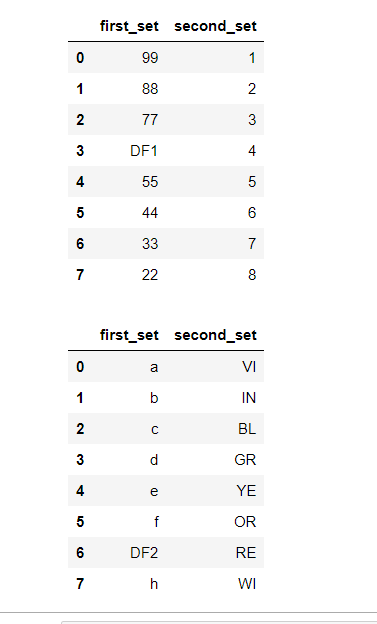Example 6: In this example, we had created another data frame ‘df2’ and ‘df3’ to replace the value of ‘df1’. Here the value of “df2” “df3” will be passed through the update method then it will find the matched entity present in “df1” after finding it will update the value with “df2” “df3” values. Pandas df.update() function to update the values identified the passed indexed in the given Series object.

Syntax: df.update(other)

Parameter :

• other: series

## Python3

 `import` `pandas as pd`   `# Creating dataframe` `df1 ``=` `pd.DataFrame([[``"rumul"``, ``10``, ``12``, ``10``],` `                    ``[``"rahul"``, ``10``, ``11``, ``16``],` `                    ``[``"purvi"``, ``18``, ``14``, ``10``],` `                    ``[``"ravi"``, ``20``, ``13``, ``30``]],` `                   ``columns``=``[``"Name1"``, ``"Maths"``,` `                            ``"Physics"``,` `                            ``"Chemistry"``])`   `# setting name1 as index column` `df1 ``=` `df1.set_index(``'Name1'``)`   `display(df1)`   `# Creating another dataframe for` `# replacement` `df2 ``=` `pd.DataFrame(` `  ``[[``"rahul"``, ``1``, ``1``]],` `  ``columns``=``[``"Name2"``, ``"Maths"``, ``"Physics"``])`   `df3 ``=` `pd.DataFrame(` `  ``[[``"purvi"``, ``5``, ``8``]],` `  ``columns``=``[``"Name3"``, ``"Maths"``, ``"Chemistry"``])`   `# setting name2 and name3 as` `# index column` `df2 ``=` `df2.set_index(``'Name2'``)` `df3 ``=` `df3.set_index(``'Name3'``)`   `# update the values at the ` `# passed index` `df1.update(df2)` `df1.update(df3)`   `display(df1)`

Output: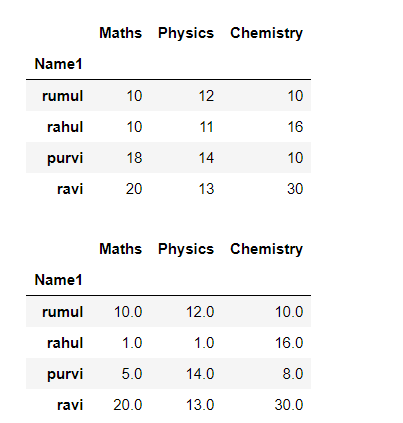Example 7: Use of isin method to filter the df and assign the desired row values. Here we selected the common ‘Name’ to filter out data from DataFrame(df1) and DataFrame(df2) after that we replaced it with the value of ‘df2’. for example, rumul’marks are replaced with 5 to 18 marks, rahul’marks are replaced with 20 to 19 marks, etc. Pandas isin() method is used to filter data frames. This method helps in selecting rows with having a particular value in a particular column.

Syntax: DataFrame.isin(values)

Parameters:

• values: iterable, Series, List, Tuple, DataFrame or dictionary.

## Python3

 `import` `pandas as pd`   `# Creating dataframe` `df1 ``=` `pd.DataFrame({``'Name'``: [``'rumul'``, ``'rahul'``,` `                             ``'ravi'``, ``'imran'``], ` `                    ``'Marks'``: [``5``, ``20``, ``8``, ``12``]})`   `df2 ``=` `pd.DataFrame({``'Name'``: [``'rumul'``, ``'rahul'``,` `                             ``'purvi'``, ``'ravi'``, ` `                             ``'imran'``],` `                    ``'Marks'``: [``18``, ``19``, ``13``, ``11``, ``15``]})`   `display(df1)` `display(df2)`   `# replace values of one DataFrame with ` `# the value of another DataFrame` `df1[``'Marks'``] ``=` `df2[df2[``'Name'``].isin(df1[``'Name'``])][``'Marks'``].values`   `display(df1)`

Output: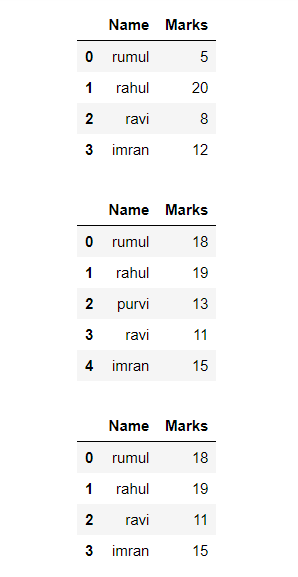Whether you're preparing for your first job interview or aiming to upskill in this ever-evolving tech landscape, GeeksforGeeks Courses are your key to success. We provide top-quality content at affordable prices, all geared towards accelerating your growth in a time-bound manner. Join the millions we've already empowered, and we're here to do the same for you. Don't miss out - check it out now!

Previous
Next